Click to Chat

1800-1023-196

+91-120-4616500

CART 0

• 0

MY CART (5)

Use Coupon: CART20 and get 20% off on all online Study Material

ITEM
DETAILS
MRP
DISCOUNT
FINAL PRICE
Total Price: Rs.

There are no items in this cart.
Continue Shopping• Complete JEE Main/Advanced Course and Test Series
• OFFERED PRICE: Rs. 15,900
• View Details

```Revision Notes on Linear Equations in One Variable

Algebraic Expressions

Any expression involving constant, variable and some operations like addition, multiplication etc is called Algebraic Expression.A variable is an unknown number and generally, it is represented by a letter like x, y, n etc.

Any number without any variable is called Constant.

A number followed by a variable is called Coefficient of that variable.

A term is any number or variable separated by operators.

Equation

A statement which says that the two expressions are equal is called Equation.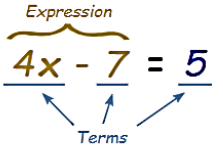Linear Expression

A linear expression is an expression whose highest power of the variable is one only.

Example

2x + 5, 3y etc.

The expressions like x2 + 1, z2 + 2z + 3 are not the linear expressions as their highest power of the variable is greater than 1.

Linear Equations

The equation of a straight line is the linear equation. It could be in one variable or two variables.

Linear Equation in One Variable

If there is only one variable in the equation then it is called a linear equation in one variable.

The general form is

ax + b = c, where a, b and c are real numbers and a ≠ 0.

Example

x + 5 = 10

y – 3 = 19

These are called linear equations in one variable because the highest degree of the variable is one and there is only one variable.

Some Important points related to Linear Equations

There is an equality sign in the linear equation. The expression on the left of the equal sign is called the LHS (left-hand side) and the expression on the right of the equal sign is called the RHS (right-hand side).

In the linear equation, the LHS is equal to RHS but this happens for some values only and these values are the solution of these linear equations.

Graph of the Linear Equation in One Variable

We can mark the point of the linear equation in one variable on the number line.

x = 2 can be marked on the number line as follows-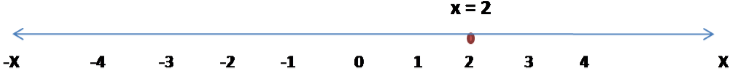Solving Equations which have Linear Expressions on one Side and Numbers on the other Side

There are two methods to solve such type of problems-

1. Balancing Method

In this method, we have to add or subtract with the same number on both the sides without disturbing the balance to find the solution.

Example

Find the solution for 3x – 10 = 14

Solution

Step 1: We need to add 10 to both the sides so that the numbers and variables come on the different sides without disturbing the balance.

3x – 10 +10 =10+14

3x = 24

Step 2: Now to balance the equation, we need to divide by 3 into both the sides.

3x/3 = 24/3

x = 8

Hence x = 8 is the solution of the equation.

We can recheck our answer by substituting the value of x in the equation.

3x – 10 = 14

3(8) – 10 = 14

24-10 = 14

14 = 14

Here, LHS = RHS, so our solution is correct.

2. Transposing Method

In this method, we need to transpose or transfer the constants or variables from one side to another until we get the solution. When we transpose the terms the sign will get changed.

Example

Find the solution for 2z +10 = 4.

Solution

Step 1: We transpose 10 from LHS to RHS so that all the constants come in the same side.

2z = 4 -10 (sign will get changed)

2z = -6

Step 2: Now divide both the sides by 2.

2z/2 = - 6/2

z = - 3

Here z = -3 is the solution of the equation.

Some Applications of Linear Equation

We can use the concept of linear equations in our daily routine also. There are some situations where we need to use the variable to find the solution. Like,

What number should be added to 23 to get 75?

If the sum of two numbers is 100 and one of the no. is 63 then what will be the other number?

Example

What is the height of the rectangle whose perimeter is 96 cm2 and the length is 12 cm?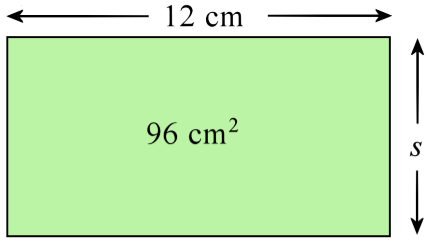Solution

Let the height of the rectangle be ‘s’.

Area of rectangle = Length × Breadth

96 = S × 12

Now, this is a linear equation with variable s.

We need to divide both sides by 12 to find the solution.

96/12 = 12s/12

s = 8

Hence the height of the rectangle is 8 cm.

Solving Equations having the Variable on both Sides

As the equation can have the variable on both the sides also so we should know how to solve such problems.

In this type of problems, we need to bring all the constants on one side and all the terms having variables on the other side. Then they can be solved easily.

Example

Find the solution of 2x−3 = 6 − x.

Solution

Step 1: Bring all the terms including variable x on LHS and the constants on the RHS.

2x + x = 6 + 3 (sign will change while changing the position of the terms)

Step 2: Solve the equation

3x = 9

Step 3: Divide both the sides by 3 to get the solution.

3x/3 = 9/3

x = 3

Hence the solution of the equation is x = 3.

Some More Applications

Example

Renu’s age is four times that of her younger brother. Five years back her age was 9 times her brother’s age. Find their present ages.Solution

Let the Renu’s brother age = x

Renu’s age = 4x (as her age is 4 times that of her younger brother)

Five years back her age was = 9(x – 5) which is equal to 4x – 5

9(x – 5) = 4x – 5

9x – 45 = 4x – 5

9x – 4x = – 5 + 45 (by transferring the variable and constants on different sides)

5x = 40

x = 40/5 = 8

Renu’s brother age = x = 8 years

Renu’s age = 4x = 4(8) = 32 years.

Reducing Equations to Simpler Form

When linear equations are in fractions then we can reduce them to a simpler form by-

Taking the LCM of the denominator

Multiply the LCM on both the sides, so that the number will reduce without the denominator and we can solve them by the above methods.

Example
Solve the linear equationSolution
As the equation is in complex form, we have to reduce it into a simpler form.

Step 1: Take the L.C.M. of the denominators, 2, 3, 4, and 5, which is 60.
Step 2: Multiply both the sides by 60,30x −12 = 20x + 15 + 60

Step 3. Bring all the variables on the LHS and all the constants on the RHS

30x − 20x = 15 + 12 + 60

10x = 87

Step 4: Dividing both the sides by 10

x = 8.7

Equations Reducible to the Linear Form

Sometimes there are some equations which are not linear equations but can be reduced to the linear form and then can be solved by the above methods.

Example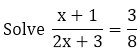Solution

This is not a linear equation but can be reduced to linear form

Step 1: Multiply both the sides by (2x + 3).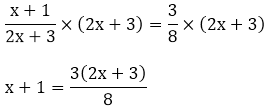Now, this is a linear equation.

Step 2: Multiply both the sides by 8.

8(x + 1) = 3(2x + 3)

8x + 8 = 6x + 9

8x - 6x = 9 - 8

2x = 1

x = 1/2

Hence the solution for the equation is x = 1/2.
```### Course Features

• 728 Video Lectures
• Revision Notes
• Previous Year Papers
• Mind Map
• Study Planner
• NCERT Solutions
• Discussion Forum
• Test paper with Video Solution